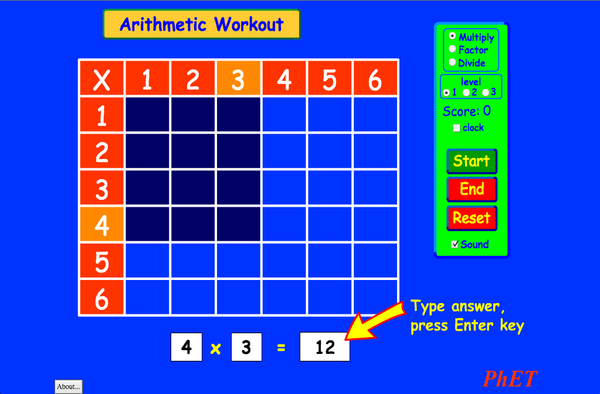# ArithmétiqueTéléchargementIntégrer Fermer Intégrer une copie fonctionnelle de cette simulation Utiliser ce code HTML pour intégrer une copie fonctionnelle de cette simulation. Vous pouvez changer la largeur et la hauteur de la simulation intégrée en modifiant les attributs "width" and "height" du HTML. Intégrer une image qui lancera la simulation en cliquant
Cliquer pour lancer
Utiliser ce code HTML pour afficher une capture d'écran avec les mots "Cliquer pour lancer" Multiplication PhET est soutenu paret des enseignants tels que vous.Retour à la version en HTML5

### Sujets

• Multiplication

### Description

Vous souvenez-vous de vos tables de multiplication?Entraînez-vous sur vos multiplications, divisions, et aptitudes à factoriser avec ce jeu passionnant. Pas de calculatrices autorisées!

### Echantillon d'objectifs d'apprentissage

• Expliquer comment les tables de multiplications aident à comprendre les multiplications, la factorisation et la division.
• Accroître la précision pour multiplier, factoriser et diviser.
• Développer des stratégies multiples pour les problèmes arithmétiques

### Alignements standards

#### Common Core - Math

3.OA.A.1
Interpret products of whole numbers, e.g., interpret 5 × 7 as the total number of objects in 5 groups of 7 objects each. For example, describe a context in which a total number of objects can be expressed as 5 × 7.
3.OA.A.2
Interpret whole-number quotients of whole numbers, e.g., interpret 56 ÷ 8 as the number of objects in each share when 56 objects are partitioned equally into 8 shares, or as a number of shares when 56 objects are partitioned into equal shares of 8 objects each. For example, describe a context in which a number of shares or a number of groups can be expressed as 56 ÷ 8.
3.OA.A.4
Determine the unknown whole number in a multiplication or division equation relating three whole numbers. For example, determine the unknown number that makes the equation true in each of the equations 8 × ? = 48, 5 = _ ÷ 3, 6 × 6 = ?
3.OA.B.5
Apply properties of operations as strategies to multiply and divide.2 Examples: If 6 × 4 = 24 is known, then 4 × 6 = 24 is also known. (Commutative property of multiplication.) 3 × 5 × 2 can be found by 3 × 5 = 15, then 15 × 2 = 30, or by 5 × 2 = 10, then 3 × 10 = 30. (Associative property of multiplication.) Knowing that 8 × 5 = 40 and 8 × 2 = 16, one can find 8 × 7 as 8 × (5 + 2) = (8 × 5) + (8 × 2) = 40 + 16 = 56. (Distributive property.)
3.OA.B.6
Understand division as an unknown-factor problem. For example, find 32 ÷ 8 by finding the number that makes 32 when multiplied by 8.
3.OA.C.7
Fluently multiply and divide within 100, using strategies such as the relationship between multiplication and division (e.g., knowing that 8 × 5 = 40, one knows 40 ÷ 5 = 8) or properties of operations. By the end of Grade 3, know from memory all products of two one-digit numbers.
3.OA.D.9
Identify arithmetic patterns (including patterns in the addition table or multiplication table), and explain them using properties of operations. For example, observe that 4 times a number is always even, and explain why 4 times a number can be decomposed into two equal addends.
Version 2.03

### Conseils des enseignantsPrésentation des contrôles des sims, simplifications des modèles, et un aperçu de la réflexion des élèves ( PDF ).

### Activités soumises aux enseignants

Juego de Aritmética (Basado en Indagación)Trish Loeblein, traducido por Diana López Lyc
Clg
Guidée
Dv
Mathématiques
How do PhET simulations fit in my middle school program?Sarah Borenstein Clg Autre Physique
Science de la Terre
Chimie
Biologie
MS and HS TEK to Sim Alignment Elyse Zimmer Lyc
Clg
Autre Chimie
Physique
Biologie
Math facts - Using the Multiplication Chart Tapan Sarkar Clg Labo Mathématiques
Back to the Basics Nyetta Abernathy Lyc
Clg
Cm2
Dv
Labo
Mathématiques
Discovering divisibility rules Stacy Larson Clg
Lyc
Labo Mathématiques
곱셈 나눗셈 연습 SIM 사용설명서 이화국(Wha Kuk Lee) Cm2
Clg
Démo
QC
Dv
Mathématiques
Physique
ARITHMETIC_DIVISON Arlete Cabral Cm2 Labo Mathématiques
OBJETO DE APRENDIZAGEM: ARITHMETIC Arlete Moura de Oliveira Cabral e Maria Cleide da Silva Barroso Cm2 Guidée Mathématiques
Operações Aritméticas Fundamentais Georgyana Cidrão; Francisco Régis Vieira Alves Cm2 Guidée
Labo
Mathématiques
Langues Télécharger ou exécuter Conseils
Afrikaans Tout AfrikaansRekenkunde
Albanais Tout shqipAritmetika
Allemand Tout DeutschRechnen
Amharique Tout Amharicየሒሳብ ስሌቶች
Anglais Tout EnglishArithmetic
Arabe Tout العربيةarithmetic
Arabe, Arabie saoudite Tout العربية (السعودية)arithmetic
Basque Tout EuskaraAritmetika
Bosniaque Tout BosanskiARITMETIKA
Chinois (Simplifié) Tout 中文 (中国)算术
Chinois (Traditionnel) Tout 中文 (台灣)算數
Coréen Tout 한국어곱셈 나눗셈 연습
Croate Tout hrvatskiAritmetika
Danois Tout DanskAritmetisk
Espagne(Perou) Tout español (Perú)Aritmética
Espagnol Tout españolAritmética
Espagnol (Mexique) Tout español (México)Aritmética
Estonien Tout EestiAritmeetika
Georgien Tout ქართულიარითმეტიკა
Grec Tout ΕλληνικάΑριθμητικός
Hébreu Tout עבריתחשבון
Hongrois Tout magyarSzorzótábla játék
Indonésien Tout Bahasa IndonesiaAritmatika
Italien Tout italianotabelline
Japonais Tout 日本語計算
Kazakh Tout KazakhАрифметика
Letton Tout LatviešuAritmētika
Macédonien Tout македонскиАРИТМЕТИКА
Marathi Tout मराठीअंकगणित
Néerlandais Tout NederlandsRekenen
Perse Tout فارسیحساب
Polonais Tout polskiArytmetyka
Portugais (Brésil) Tout português (Brasil)Aritmética
Roman Tout românăAritmetica
Russe Tout русскийАрифметика
Serbe Tout СрпскиАРИТМЕТИКА
Slovaque Tout SlovenčinaAritmetika
Suédois Tout svenskaMatteräkning
Swahili Tout SwahiliHesabu
Turc Tout TürkçeAritmetik
Turkmène Tout TurkmenArifmetika
Vietnamien Tout Tiếng ViệtSố học
Windows Macintosh Linux
Microsoft Windows
XP/Vista/7/8.1/10
Macromedia Flash 9 or later
OS X 10.9.5 or later
Macromedia Flash 9 or later
Macromedia Flash 9 or later
Equipe de design Librairies tierces Remerciements à :